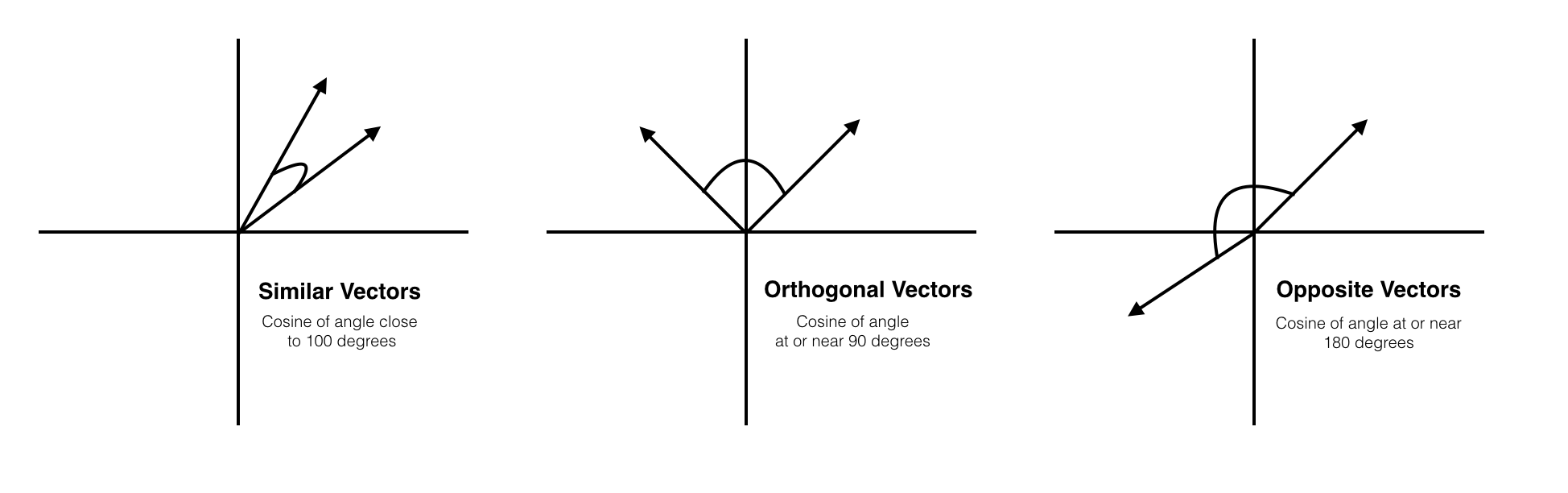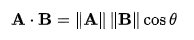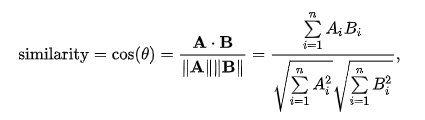# Cosine Similarity

## What is Cosine Similarity?

Cosine Similarity measures the cosine of the angle between two non-zero vectors of an inner product space. This similarity measurement is particularly concerned with orientation, rather than magnitude. In short, two cosine vectors that are aligned in the same orientation will have a similarity measurement of 1, whereas two vectors aligned perpendicularly will have a similarity of 0. If two vectors are diametrically opposed, meaning they are oriented in exactly opposite directions (i.e. back-to-back), then the similarity measurement is -1. Often, however, Cosine Similarity is used in positive space, between the bounds 0 and 1. Cosine Similarity is not concerned, and does not measure, differences is magnitude (length), and is only a representation of similarities in orientation.## How does Cosine Similarity Work?

The Cosine Similarity measurement begins by finding the cosine of the two non-zero vectors. This can be derived using the Euclidean dot product formula which is written as:Then, given the two vectors and the dot product, the cosine similarity is defined as:The output will produce a value ranging from -1 to 1, indicating similarity where -1 is non-similar, 0 is orthogonal (perpendicular), and 1 represents total similarity.

## Applications of Cosine Similarity

Cosine Similarity has applications that extend beyond abstract mathematics. The measurement is used in processes of data mining, information retrieval, and text matching. Once the vectors are assigned to properties of a variable, the measurement becomes a valuable tool for understanding similarities between objects.

### Cosine Similarity and Machine Learning

Machine learning uses Cosine Similarity in applications such as data mining and information retrieval. For example, a database of documents can be processed such that each term is assigned a dimension and associated vector corresponding to the frequency of that term in the document. This allows for a Cosine Similarity measurement to distinguish and compare documents to each other based upon their similarities and overlap of subject matter.